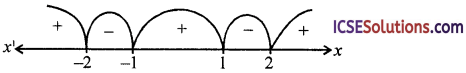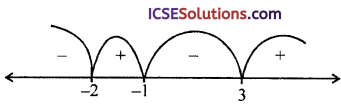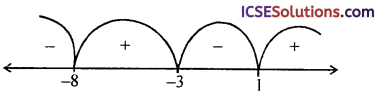Interactive ISC Mathematics Class 12 Solutions Chapter 7 Applications of Derivatives Ex 7.4 engage students in active learning and exploration.

## ML Aggarwal Class 12 Maths Solutions Section A Chapter 7 Applications of Derivatives Ex 7.4

Question 1.
Solve for x :
(i) x (x – 2) (x – 5) (x + 3) > 0
(ii) x4 – 5x2 + 4 ≥ 0.
Solution:
(i) Given, x (x – 2) (x – 5) (x + 3) = 0 ………….(1)
Here, mark the points – 3, 0, 2, 5 on real line.
By method of intervals, the inequality (1) is satisfied when
x < – 3 or 0 < x < 2 or x > 5∴ The required solution set is (- ∞, – 3) ∪ (0, 2) ∪ (5, ∞).

(ii) Given inequality be x4 – 5x2 + 4 ≥ 0
(x2 – 1) (x2 – 4) ≥ 0
⇒ (x- 1) (x – 2) (x + 1) (x + 2) ≥ 0 ………….(1)
Here mark the real numbers – 1, – 2, 1, 2 on real line and by using method of intervals, eqn. (1) is satisfied
When x ≤ – 2 or – 1 ≤ x ≤ 1 or x ≥ 2
∴ required solution set be (- ∞, – 2] ∪ [- 1, 1] ∪ [2, ∞)Question 2.
Find all real values of x which satisfy
(i) x3 (x – 1) (x – 2) > 0
(ii) x2 (x – 1) (x – 2) ≤ 0
Solution:
(i) Given inequality be x3 (x – 1) (x – 2) > 0
⇒ x (x – 1) (x – 2) > 0 ……….(1)
[∵ x2 ≥ 0]
Mark the real numbers 0, 1, 2 on real line.
Using method of intervals, eqn. (1) is satisfied when 0 < x < 1 or x > 2
∴ required solution set be given by (0, 1) ∪ (2, ∞).(ii) Given inequality be
x2 (x – 1) (x – 2) ≤ 0
(x – 1) (x – 2) ≤ 0 ……….(1)
[∵ x2 ≥ 0]
Mark the numbers 1, 2 on real line and by using method of intervals, eqn. (1) is satisfied when 1 ≤ x ≤ 2
also given eqn. is satisfied when x = 0
∴ required solution set = [1, 2] ∪ {0}Question 3.
(i) $$\frac{1}{x-2}$$ ≤ 1
(ii) $$\frac{(x+1)(x-3)}{x+2}$$ ≥ 0
Solution:
(i) Given inequality be $$\frac{1}{x-2}$$ ≤ 1 ……………(1); x ≠ 2
Since (x – 2)2 > 0 ∀ x ∈ R, x ≠ 2
⇒ (x – 2) ≤ (x – 2)2
[multiplying by (x – 2)2]
⇒ (x – 2)2 – (x – 2) ≥ 0
⇒ (x – 2) (x – 3) ≥ 0
Mark the numbers 2, 3 on real line.
Using method of intervals, eqn. (2) is satisfied when x ≤ 2 or x ≥ 3But x ≠ 2.
Thus, required solution set be (- ∞, 2) ∪ [3, ∞)

(ii) Given inequality $$\frac{(x+1)(x-3)}{x+2}$$ ≥ 0 ; x ≠ – 2
∴ (x + 2)2 > 0 ∀ x ∈ R, x ≠ – 2
⇒ (x + 1) (x – 3) (x + 2) ≥ 0 ………..(1)
[Multiplying both sides by (x + 2)2 > 0]
Mark the numbers – 1, – 2, 3 on real line and by using method of intervals, eqn. (1) is satisfied
When – 2 ≤ x ≤ – 1 or x ≥ 3
But x ≠ – 2∴ required solution is given by (- 2, -1] ∪ [3, ∞).Question 4.
Find the values of x for which:
(i) $$\frac{x^2+6 x-11}{x+3}$$
(ii) $$\frac{x^2-3 x+24}{x^2-3 x+3}$$ < 4
Solution:
(i) Given inequality be $$\frac{x^2+6 x-11}{x+3}$$ < – 1
⇒ $$\frac{x^2+6 x-11}{x+3}$$ + 1 < 0
⇒ $$\frac{x^2+7 x-8}{x+3}$$ < 0
⇒ $$\frac{(x-1)(x+8)}{x+3}$$ < 0 ;
Since (x + 3)2 > 0 ∀ x ∈ R, x ≠ – 3
(x – 1) (x + 8) (x + 3) < 0
[multiplying by (x + 3)2]
Mark the numbers – 3, – 8 and 1 on real line.
Using methods of intervals, given inequality satisfied when– 3 < x < 1 or x < – 8
∴ required solution set be (- ∞, – 8) ∪ (- 3, + 1).(ii) Given $$\frac{x^2-3 x+24}{x^2-3 x+3}$$ < 4∴ from (1) ;
– 3 (x2 – 3x – 4) < 0
⇒ (x – 3x – 4) > 0
⇒ (x + 1) (x – 4) > 0 ………….(2)
Mark the real numbers – 1 and 4 on real line.
Using method of intervals, eqn. (2) is satisfied when x < – 1 or x > 4
∴ required solution set be (- ∞, – 1) ∪ (4, ∞).